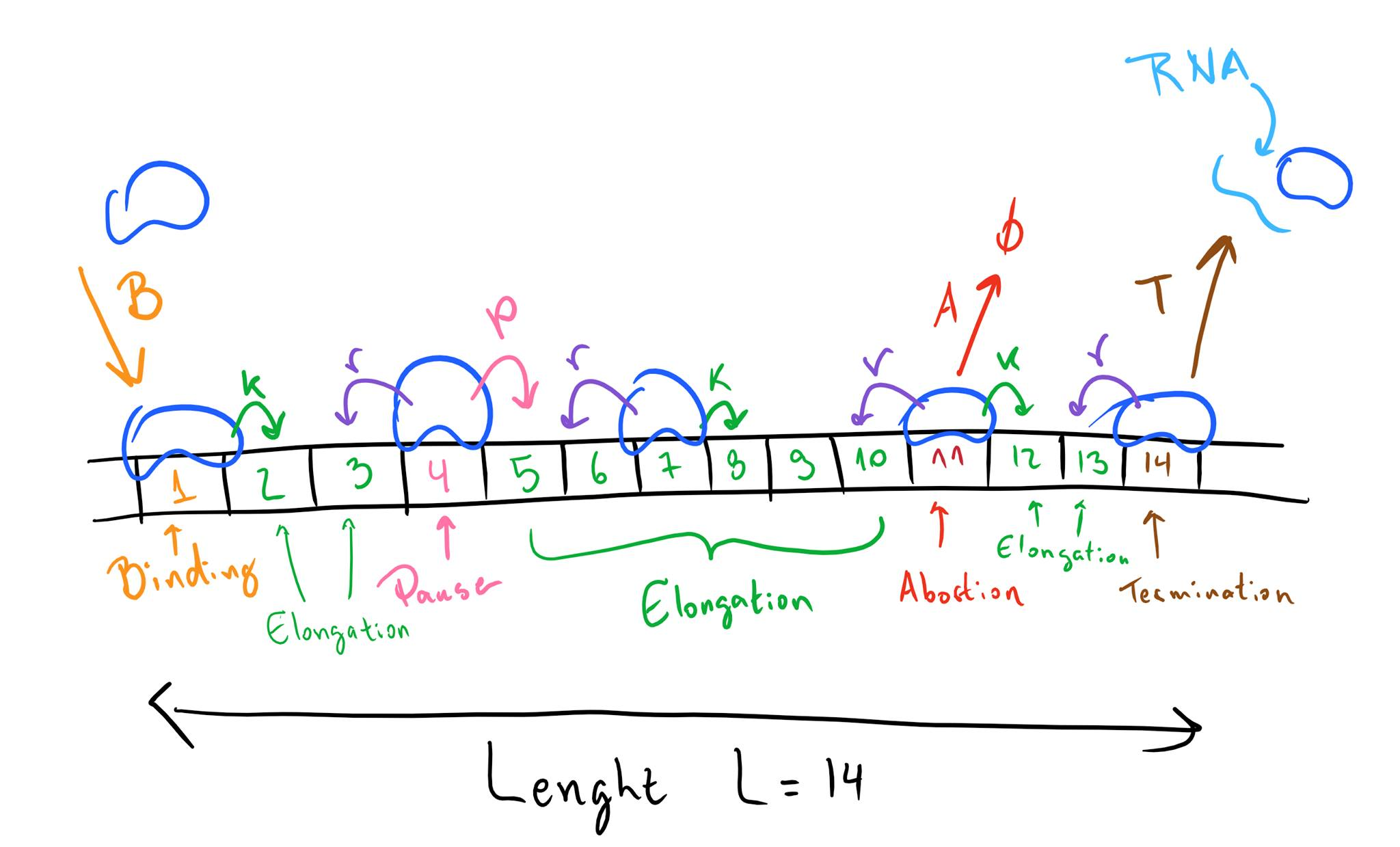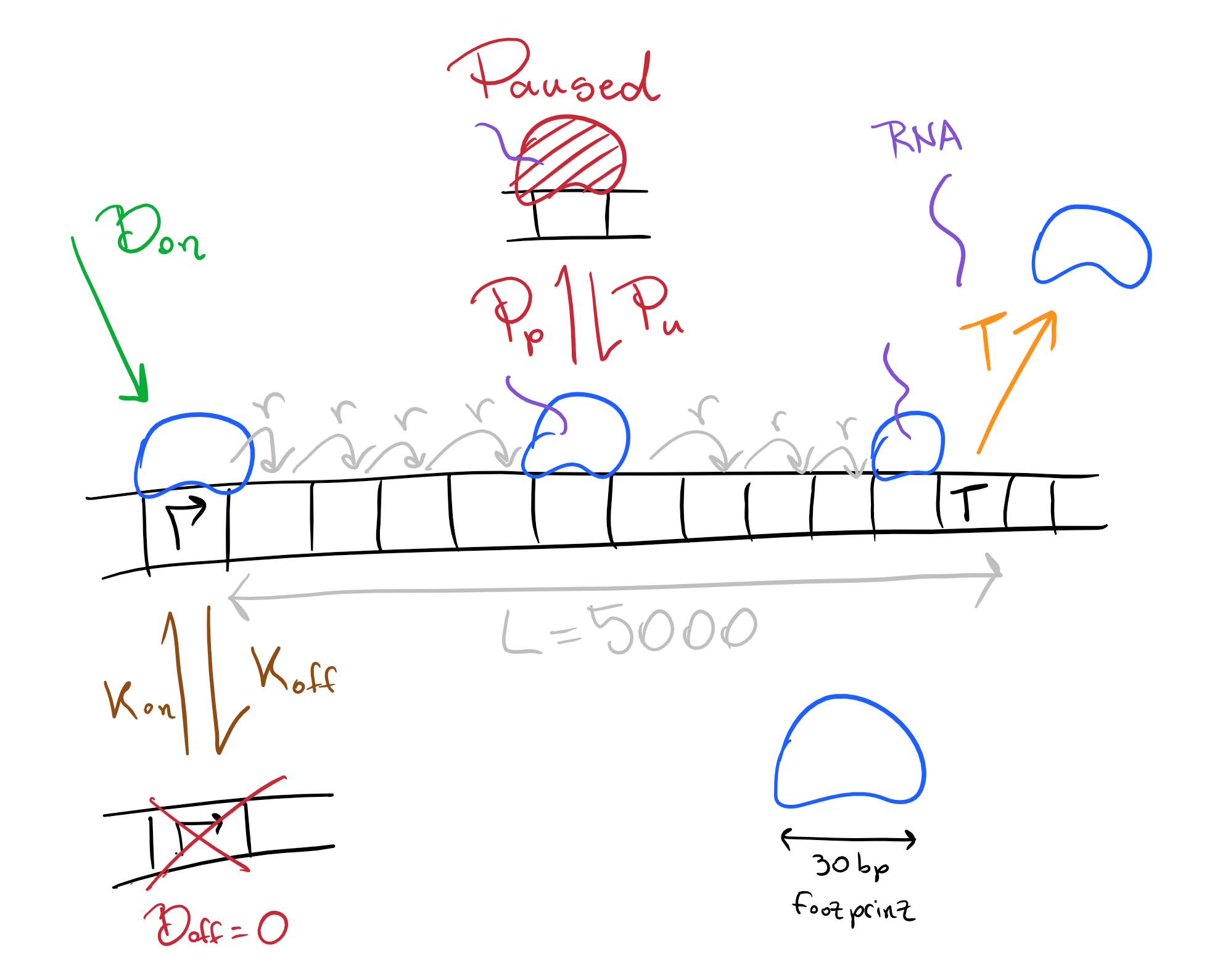Skip to content
{{ message }}

# Munfred / genesimulator

Python scripts for an easy to use, modular and customizable gene simulator using the Gillespie algorithm.

Switch branches/tags
Nothing to show

## Files

Failed to load latest commit information.
Type
Name
Commit time

# genesimulator

Python scripts for an easy to use, modular and customizable gene simulator using the Gillespie algorithm.

Users can model gene architectures by changing 5 rates for each gene locus: elongation, backtracking, binding, termination and abortion. The program output is a graphical representation of gene occupancy over time, a histogram of time interval between consecutive terminations, a histogram of the time taken per polymerase to transcribe the gene, and a graph of total terminated polymerases.

For details on the math see the genesimulator guide

Note there are two scripts you can use: `genesimulator_simple.py` or `genesimulator_bistable.py` which has extra features described in the last section

## Modeling Framework

Instead of considering a certain number of base pairs between each polymerase, we instead consider that the smallest stretch of DNA we can simulate is as long as a the polymerase footprint.So the smallest 'hop' that the polymerase perform is equal to it's footprint, which is about 40 base pairs. This should not affect simulation results, but considerably simplifies the model and minimizes the necessary computation.

We consider genes as unidimensional lattices of length L where each locus in the lattice correspond to a stretch of DNA the size of the polymerase footprint. Each position may be either empty or be occupied by one polymerase at a time.

Each locus takes 5 parameters for the different possible events:

Elongation rate `k` If the locus is occupied by a polymerase, it can take a step forward, freeing its location and occupying the next one.

Backtrack rate `r` If the locus is occupied by a polymerase, it can take a step back, freeing its location and occupying the previous one.

Binding rate `B` If the locus is free, a new polymerase may bind to it.

Termination rate `T` If the locus is occupied by a polymerase, it can become unoccupied, and an RNA transcript is produced.

Abortion rate `A` If the locus is occupied by a polymerase, it can become unoccupied, but no RNA transcript is produced.

The rates define how many "events per unit of time" happen on average. They are all treated as simple biochemical reaction steps, and have an exponential probability distribution over time. The concatenation of several such simple steps leads to different resulting probability distributions.Example gene we could build with 14 Loci. In it, locus 1 is a binding element, where polymerase can bind with rate `B`, and elongate with rate `k`. The backtrack, abortion and termination rates are all zero on locus 1. On loci 2 to 3, 5 to 10 and 12 to 13, it can elongate with rate `k`, or backtrack with rate `r`, while all other rates are zero. We could say that those are 3 elongation elements of length 2, 5 and 6 respectively. Locus 4 is also an elongation element with backtrack rate `r`, but with elongation rate `p`. If we consider `p < k`, then locus 4 would be a pausing element, where polymerase takes a longer time than normal to advance. On locus 11, in addition to normal elongation rates, the polymerase may abort with rate A, and so this is an abortion element. Finally on locus 14 it can either backtrack with rate r, or terminate with rate T and produce an RNA transcript. Note that it cannot elongate (the elongation rate is 0), this is the end of our gene.

## `genesimulator_bistable`

`genesimulator_bistable.py` includes:

• Bistable promoter which can switch between ON/OFF states
• Polymerase can go into paused state
• Polymerase have footprints in bp

For `genesimulator_bistable.py` the simulation now is considerably longer so the simulator outputs a CSV file with gene occupancy and a txt file with other parameters instead of plotting them.

Some examples of biological rates:

``````Footprint of 30 base pairs
B = 0.5            polymerase binding rate on the ON state (zero for OFF state)
r = 80               elongation rate after first escape step
k_off = 0.2       rate at which the an OFF promoter goes to the ON state
k_on = 0.5       rate at which the an ON promoter goes to the OFF state
k_p- = 4           rate for an paused polymerase exit the paused state
k_p+ = 0.01     rate at which an elongating  polymerase goes to the paused state
``````## About

Python scripts for an easy to use, modular and customizable gene simulator using the Gillespie algorithm.

## Releases

No releases published

## Packages 0

No packages published